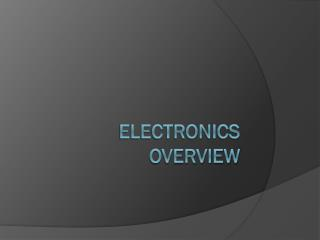DownloadDownload PresentationElectronics Overview

# Electronics Overview

Download Presentation## Electronics Overview

- - - - - - - - - - - - - - - - - - - - - - - - - - - E N D - - - - - - - - - - - - - - - - - - - - - - - - - - -
##### Presentation Transcript

1. ElectronicsOverview

2. Terms Ohm The ohm (symbol: Ω) is the SI unit of**electrical impedance or, in the direct current case, electrical resistance, named after Georg Simon Ohm.

3. Ohm's law is one of the most important concepts in electronics. Fortunately it's only a very simple mathematical relationship between current, voltage, and resistance. According to the Ohm's law, voltage equals current times resistance which is expressed in the following equation:***E=IRwhere E = voltage, I = current, and R = resistance ***Review page 14

4. Electrical impedance, or simply impedance, describes a measure of opposition to a sinusoidal alternating current (AC). • Electrical impedance extends the concept of resistance to AC circuits, describing not only the relative amplitudes of the voltage and current, but also the relative phases. • When the circuit is driven with direct current (DC) there is no distinction between impedance and resistance; the latter can be thought of as impedance with zero phase angle. • **The symbol for impedance is usually Z. • The term impedance was coined by Oliver Heaviside in July 1886.

5. Voltage To make sense of voltage, we will need to make an analogy. Lets imagine that electrons are represented by a marble on a flat plane. At this point, the plane is level and the marble does not move. If the plane is lifted at one side, the marble will roll down to the lower point. In electricity, the high point is a point with lots of electrons and the low point is a point with a lack of electrons. The high point is called the high potential and the low point is the low potential. The difference between these two points is called the potential difference. The larger the potential difference, the larger the voltage. Voltage can be thought of as the measure of the pressure pushing the electrons. The higher the pressure, the higher the voltage. Voltage is represented by the letter E. The basic unit of measure is volts or the letter V. ***One volt will push 1 amp of current through 1 ohm of resistance.

6. Current Electric current is the amount of electrons, or charge, moving past a point every second. It is basically the speed of electron flow. The faster the electron flow, the higher the current. Current is represented by the letter I. The basic unit for measuring current is ampere. Ampere can be abbreviated to amp or just A. 1 amp = 1 coulomb/secMeaning for every amp, there are 6.25x10^18 electrons moving past a point every second. One coulomb is the amount of electric charge transported in one second by a steady current of one ampere.

7. Power is simply the amount of energy used or the amount of "work" a circuit is doing. Power is represented by the letter P. The basic unit for measuring power is watts or the letter W. To find power, all you need is a simple equation: P=EIor ***Power equals voltage times current.

8. Inductance is the property in an electrical circuit where a change in the electric current through that circuit induces an electromotive force (EMF) that opposes the change in current (See Induced EMF).

9. In electromagnetism and electronics, capacitance is the ability of a body to hold an electrical charge. • Capacitance is also a measure of the amount of electric charge stored (or separated) for a given electric potential. • A common form of charge storage device is a parallel-plate capacitor. In a parallel plate capacitor capacitance is directly proportional to the surface area of the conductor plates and inversely proportional to the separation distance between the plates.